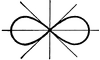# LemniscateA Lemniscate is, in general, a curve generated by a point moving so that the product of its distances from two fixed points is the square of half the distance between the points. It is a particular case of the Cassinian oval and resembles a figure 8. When the line joining the two fixed points is the axis of x and the middle point of this line is the origin, the Cartesian equation is the fourth degree equation, (((x^2)+(y^2))^2)=2(a^2)((x^2)-(y^2)). The polar equation is (ℽ^2) = 2(a^2)cos(2θ). The locus of the feet of the perpendiculars from the center of an equilateral hyperbola to its tangents is a lemniscate. The name lemniscate is sometimes given to any crunodal symmetric quartic curve having no infinite branch. The name is also sometimes given to a general class of curves derived from other curves in the way that the above is derived from the equilateral hyperbola. With these more general definitions of the lemniscate the above curve is called the lemniscate of Bernoulli.

### Galleries

Conic Sections: Hyperbolas, Analytical Geometry

### Source

TIFF (full resolution)

3119×1890, 200.3 KiB

Large GIF

1024×620, 38.8 KiB

Medium GIF

640×387, 23.2 KiB

Small GIF

320×193, 10.3 KiB